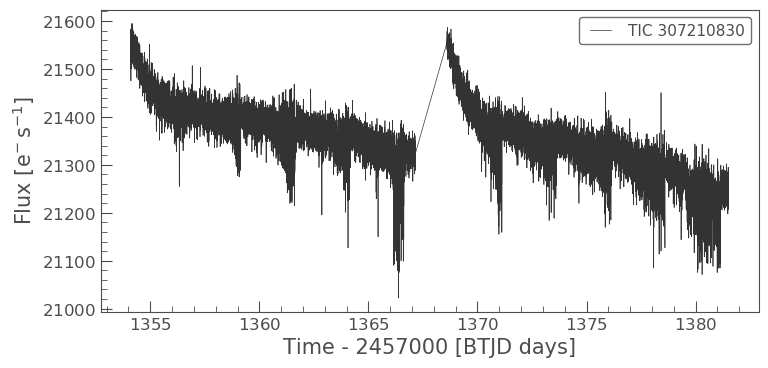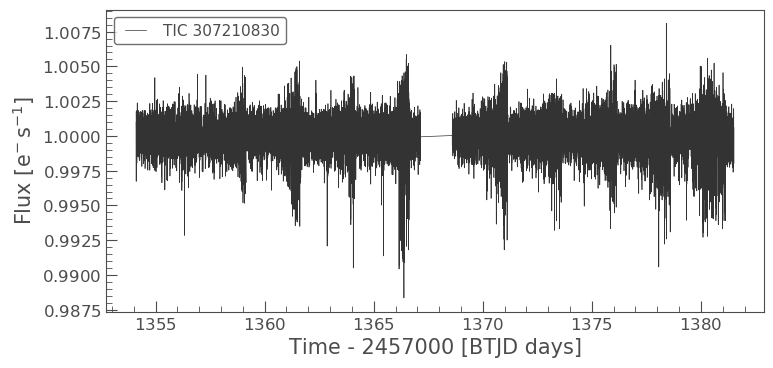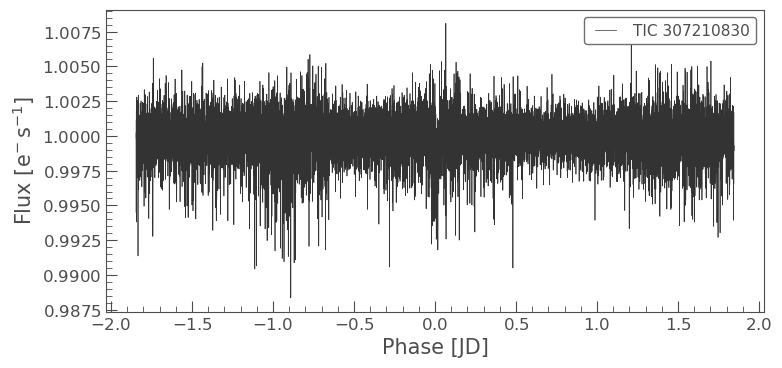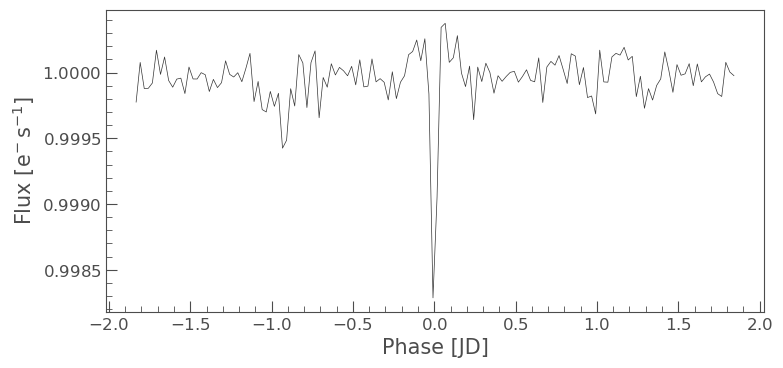# LightCurve objects

## Understanding LightCurve objects

### Learning goals

In our TargetPixelFile tutorial we learned, how to obtain data files for our objects of interest and how to examine the metadata stored in the headers.

In this tutorial we will learn the following, - How to use Lightkurve to create a TESSLightCurve object. - How to examine the object data, and understand its format. - What is simple aperture photometry (SAP). - How to plot a light curve.

### What is LightCurve object?

You can create a TESSLightCurve object from a TargetPixelFile (TPF) object using Simple Aperture Photometry (SAP).

### What is Simple Aperture Photometry (SAP)?

Aperture Photometry is the act of summing up the values of all the pixels in a pre-defined aperture as a function of time. By carefully choosing the shape of the aperture mask, you can avoid nearby contaminants or improve the strength of the specific signal you are trying to measure relative to the background.

The SAP light curve is a pixel summation time-series of all calibrated flux falling within the optimal aperture, as stored and defined in the TPF.

In this notebook we will demonstrate, let’s create a TESSLightCurve from a TESSTargetPixelFile.

### Imports

This tutorial requires that you import Lightkurve.

```%matplotlib inline
import lightkurve as lk
```

### Defining terms

• Target Pixel File (TPF): A file containing the original CCD pixel observations from which light curves are extracted.
• LightCurve Object: Obtained from a TPF and contains light curve information derived using simple aperture photometry.
• Cadence: The rate at which TESS photometric observations are stored.
• Sector: One of TESS’s 27 (to date) observing periods, approximately ~27 days in duration.
• Simple Aperture Photometry (SAP): The act of summing all pixel values in a pre-defined aperture as a function of time.

For this tutorial we will use the L 98-59 System focusing on planet c.

First we search for appropriate Target Pixel Files on MAST using the search_targetpixelfile function.

```search = lk.search_targetpixelfile('TIC 307210830 c')
search
```
SearchResult containing 7 data products.
#observationauthortarget_nameproductFilenamedistance
0TESS Sector 2SPOC307210830tess2018234235059-s0002-0000000307210830-0121-s_tp.fits0.0
1TESS Sector 5SPOC307210830tess2018319095959-s0005-0000000307210830-0125-s_tp.fits0.0
2TESS Sector 8SPOC307210830tess2019032160000-s0008-0000000307210830-0136-s_tp.fits0.0
3TESS Sector 9SPOC307210830tess2019058134432-s0009-0000000307210830-0139-s_tp.fits0.0
4TESS Sector 10SPOC307210830tess2019085135100-s0010-0000000307210830-0140-s_tp.fits0.0
5TESS Sector 11SPOC307210830tess2019112060037-s0011-0000000307210830-0143-s_tp.fits0.0
6TESS Sector 12SPOC307210830tess2019140104343-s0012-0000000307210830-0144-s_tp.fits0.0

Our object of interest can be found in seven sectors. Let’s take the first sector, sector 2 and download that.

```tpf = lk.search_targetpixelfile('TIC 307210830 c', sector=2).download()
tpf
```
```TessTargetPixelFile(TICID: 307210830)
```

### Creating and analizing the LightCurve object

Great we now have our TPF! Lets convert this TPF into a TessLightCurve object using the to_lightcurve function.

To create the SAP lightcurve we will pass an aperture_mask to the to_lightcurve function. The default is to use the SPOC pipeline aperture, which sums all the pixels in its defined mask.

```lc = tpf.to_lightcurve(aperture_mask=tpf.pipeline_mask)
lc
```
TessLightCurve targetid=307210830 length=18317
electron / selectron / spixpix
objectfloat32float32float64float64int32int32
1354.108823127242721566.34960937516.116119384765625664.6090864691554339.4764484490161911910
1354.110211988899421563.8867187516.118038177490234664.6261723169015339.46842003296774911920
1354.11298971215321475.16210937516.089221954345703664.606630403678339.4604662968742911940
1354.114378573809721583.3085937516.12527084350586664.6414481151693339.4832617761526911950
1354.115767435524321575.64062516.121679306030273664.6354584758038339.4735678477034911960
1354.117156297180421563.101562516.115528106689453664.6334974032626339.472138768046911970
1354.118545158894721552.93554687516.112627029418945664.625177003332339.46675685339096911980
1354.119934020551521532.9023437516.10567855834961664.6301979867933339.4699372207359911990
1354.121322882266721533.82812516.105731964111328664.6262018316135339.46553338843912000
.....................
1381.500103252329421262.49414062516.291688919067383664.5744500858646339.35132780163921109130
1381.501492120737821289.82812516.302898406982422664.5797804765874339.34913985203471109140
1381.502880989145821266.351562516.29288673400879664.5790106545255339.35133129076251109150
1381.504269857438221234.84570312516.279603958129883664.5730941550626339.35556313817051109160
1381.505658725846621244.95312516.281909942626953664.5782007755507339.34683164655671109170
1381.507047594255521210.757812516.267162322998047664.5770708377116339.34423590600691109180
1381.50843646254821231.0117187516.27315330505371664.5786574675517339.342172455105361109190
1381.509825330956321250.46679687516.277507781982422664.5722297003167339.35132729757531109200
1381.511214199248821236.3554687516.2720890045166664.582152318805339.34521784277111109210
1381.512603067657721265.8398437516.278945922851562664.5729270180528339.3497104930431109220

We’ve built a new TESSLightCurve object called lc. Note although we used the SPOC aperture mask you can pass your own aperture, (specified by a boolean numpy array) as seen in the Making Custom Apertures tutorial.

The above table displays all the light curve data.

TESSLightCurve objects have many useful functions that you can use. As with a TPF you can access the meta data very simply.

```lc.sector
```
```2
```

Of course you still have access to time and flux attributes. In a light curve, there is only one flux point for every cadence.

```lc.flux, lc.time
```
```(<Quantity [21566.35 , 21563.887, 21475.162, ..., 21250.467, 21236.355,
21265.84 ] electron / s>,
<Time object: scale='tdb' format='btjd' value=[1354.10882313 1354.11021199 1354.11298971 ... 1381.50982533 1381.5112142
1381.51260307]>)
```

You can also check the Combined Differential Photometric Precision (CDPP) RMS per transit duration noise metric (see Gilliland et al., 2011 for more details) of the light curve using the built in method estimate_cdpp:

```lc.estimate_cdpp()
```
300.86106  ppm

The above is the Savitzky-Golay CDPP noise metric in units parts-per-million (ppm)

### Plotting the light curve

We can now use the built in plot function on the TESSLightCurve object to plot the time series. You can pass plot any keywords you would normally pass to matplotlib.pyplot.plot.

```%matplotlib inline
lc.plot();
```### Manipulating the light curve

There are a set of useful functions in LightCurve objects which you can use to work with the data. These include: * flatten(): Remove long term trends using a Savitzky–Golay filter * remove_outliers(): Remove outliers using simple sigma clipping * remove_nans(): Remove infinite or NaN values (these can occur during thruster firings) * fold(): Fold the data at a particular period * bin(): Reduce the time resolution of the array, taking the average value in each bin.

We can use these simply on a light curve object

```flat_lc = lc.flatten(window_length=401)
flat_lc.plot();
```#### Folding the light curve

From the L 98-59 System paper we know that planet c has a time corresponding to zero phase (noted as epoch_time) of 1367.2755 BTJD days and period of 3.690621 days. We can use the fold() function to find the transit in our data.

```folded_lc = flat_lc.fold(period=3.690621,  epoch_time=1367.2755)
folded_lc.plot();
```#### Binning data

Often to see a trend it can be beneficial to bin the data, this can be achieved via the bin() function.

```binned_lc = folded_lc.bin(time_bin_size=0.025)
binned_lc.plot();
```We can now see our transit very clearly! Note that we can achieve the same plot from our data using one line of code instead of several, see below.

```lc.remove_nans().flatten(window_length=401).fold(period=3.690621,  epoch_time=1367.2755).bin(time_bin_size=0.025).plot();
```